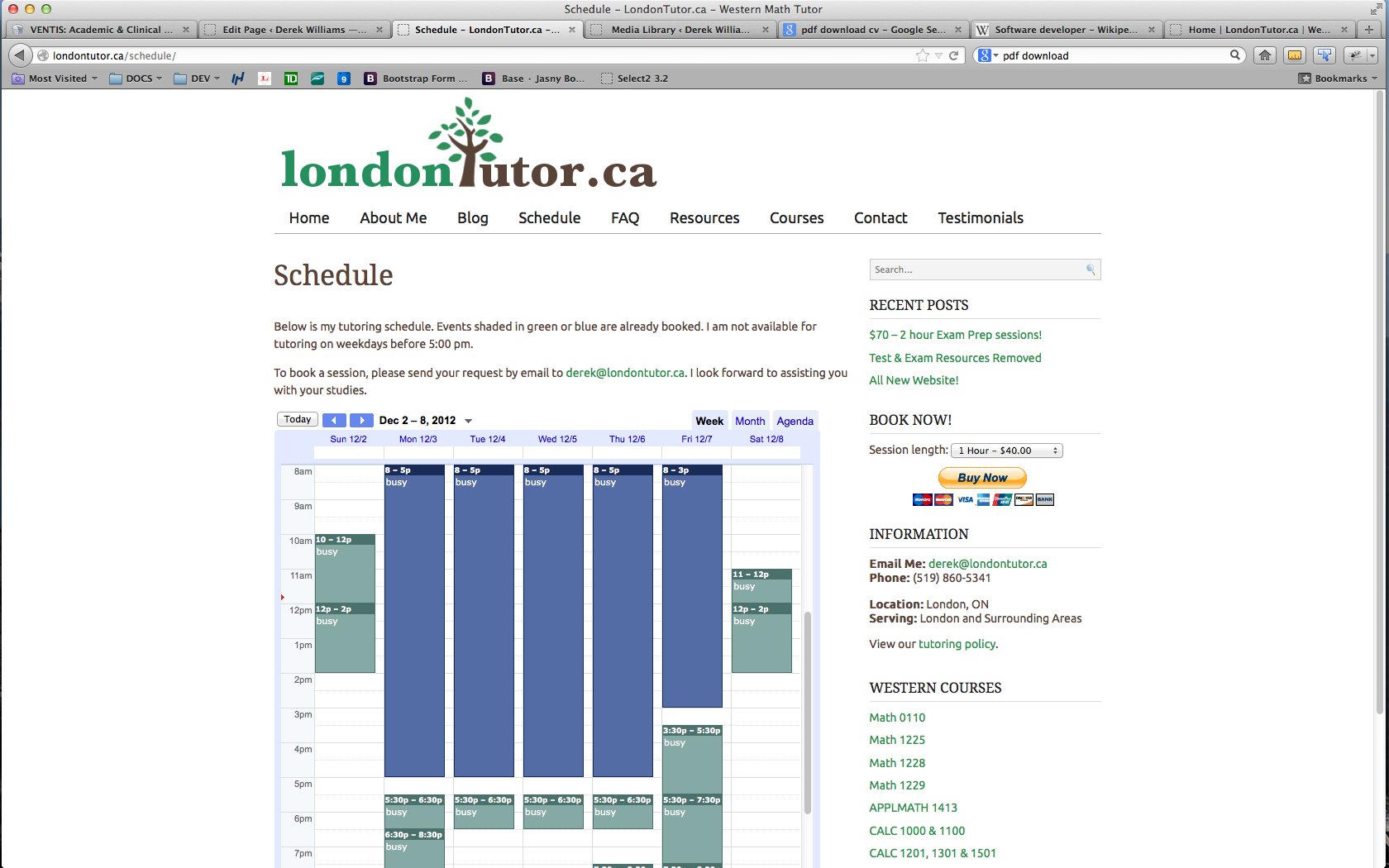London TutorTechnologies

LAMP, PHP, Apache, WordPress, jQuery, MySQL

Key responsibilities

• Founder of LondonTutor.ca
• Design & development of WordPress tutoring website
• Private tutor of Mathematics and Statistics courses at Western University
• Courses included:
• CALC1000 & CALC1301– Calculus I & Calculus II
• MATH0110 – Introduction to Calculus
• MATH1225 – Methods of Calculus
• MATH1228 – Methods of Finite Mathematics
• MATH1229 – Methods of Matrix Algebra
• MATH1600 – Linear Algebra I
• STATS1024 – Introduction to Statistics
• APPLMATH1413 – Applied Mathematics for Engineers I
• SOCIOLOGY2205 – Statistics for Sociology
• STATS2035 – Statistics for Business & Social Science
• BIOLOGY2244 – Analysis & Interpretation of Biological Data
• STATS2244 – Statistics for Science
• STATS2857 – Probability & Statistics I
Tags: , , , ,